# Overview

SurveyToGo has complete randomization support for the following:

• Randomize Sub-Chapters
• Randomize Questions
• Randomize Iterations

# A. Randomize Sub-Chapters

There are two ways to implement Sub-Chapters randomization:

1. Random order (determined by the system)

Let's look at this set of chapters and questions as an example: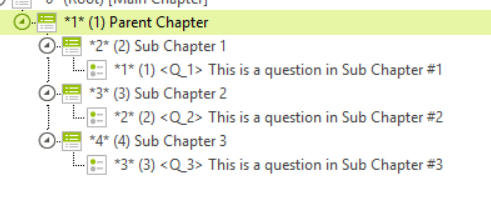As you see, chapter indexes 2, 3 and 4, are Sub-Chapters of chapter index 1 (the "Parent Chapter"). To set a random order for those Sub-Chapters, go the "Parent Chapter"'s "Advanced" tab and under the "Randomization" section enable the "Randomize Sub-Chapters" checkbox: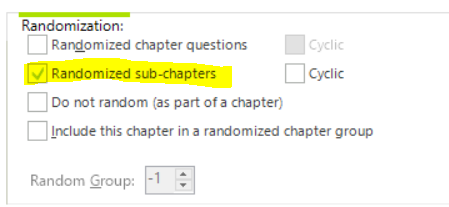2. Given order (specific order you want based on your own logic)

Randomizing sub-chapters in a given order requires some more advanced scripting technique. Please view this link to see a sample:

https://support.dooblo.net/hc/en-us/articles/208296545-How-to-Enter-X-out-of-Y-chapters

Retrieve the sub-chapter random order:

To retrieve the randomization order for the Answers use this function:
GetChaptersRandomOrder(int inChapterIndex)

# B. Randomize Questions

There are two ways to randomize questions:

1. Random order (determined by the system)

Let's look at this set of questions: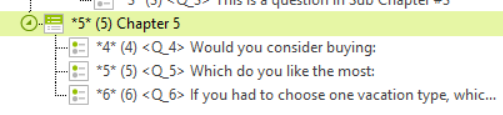And you wish to randomize the order of the questions shown. Simply:

1. Select the Chapter and switch to the “Advanced” tab: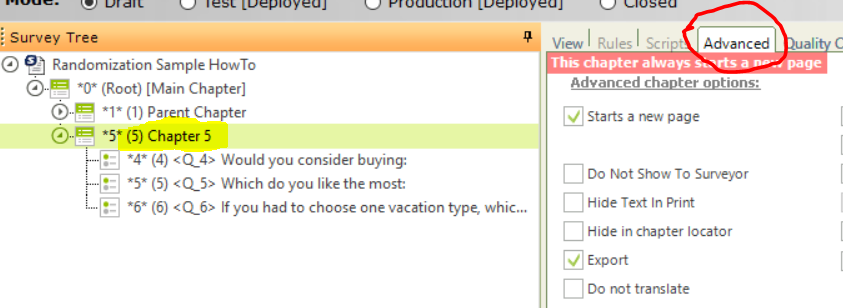2. Then, on the bottom, check the “Randomized chapters questions” box: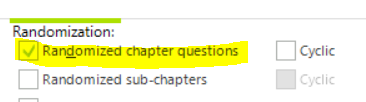If you need to have the questions randomized in a “Cyclic” method, you can check the “Cyclic” box. “Cyclic” means that while the first question is selected at random, the next questions will be the ones after the first as set in the original order.

Excluding a question from the randomized questions:

If you need to exclude one or more questions from of the randomization:

Select the relevant question and click the “Advanced” tab. Then, check the “Do not random (as part of chapter)” box: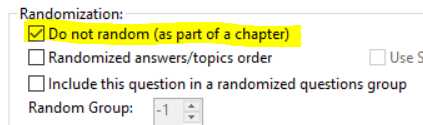Retrieve the random questions order:

To retrieve the randomization order for the questions use this function (since all the questions are randomized under a chapter you can use this code in one of the questions, there's no need to add this to each question):
GetQuestionsRandomOrder(inQuesIndex)

https://support.dooblo.net/hc/en-us/articles/360000538969-GetQuestionsRandomOrder

2. Given order (specific order you want based on your own logic)

Randomizing questions in a given order requires some more advanced scripting technique. Please view this link to see a sample:

https://support.dooblo.net/hc/en-us/articles/115000910925-How-to-control-the-execution-order-of-questions

# C. Randomize Topics / Answers

## Randomize all (or some) answers of a question

Answers randomization can be achieved with a Random order or a Given order.

Random order

There are two ways to set a random order foe answers:

1. Configuration based:

Switch to the “Advanced” tab of the question: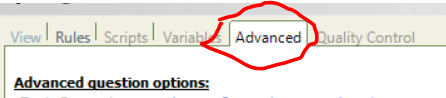On the bottom, check the “Randomized answers/topics order” box: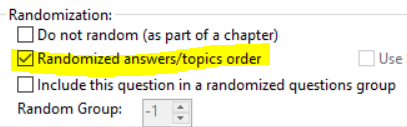Excluding an answer from the randomization:

If you need to randomize all the answers but keep one answer static, for example – in the following question, we would like to keep the last answer not randomized: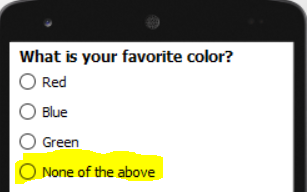To do this, follow the same steps as above to get the answers to be randomized, but in addition do the following: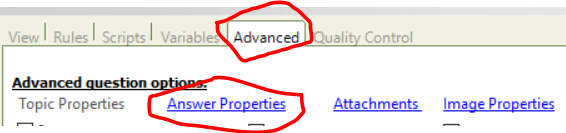2. In the answer properties screen, scroll to the right and check the “Do not Random” box of the relevant answer: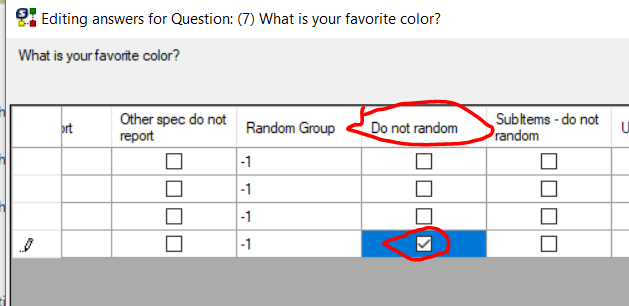3. Click the OK button.

To retrieve the randomization order for the Answers use this function:

https://support.dooblo.net/hc/en-us/articles/208296115-How-To-Use-Random-Groups

2. Script based:

The second way to randomize answers is through script (with UL code). Use this function in the question's Start script to randomize its answers:

Given order

You can randomize answers based on a given order you create, based on your specific logic. This is also script based and done with an overload of the RandomizeAnswers function, by sending it the question index and an array of the answers indexes in the random order needed (again, you can read about that overload function here) you can. For example, if I have 5 answers in the questions, this can be a valid array: [3,4,1,5,2].

NOTE: In order for the Given order randomization to work, the array must contain ALL the answers indexes that are in the question.

Keeping the same given order in multiple questions:

When randomizing answers with a given order, sometime you's want to have the exact same given order in several different questions. View this link to see how this can be done:

## Randomize Topics of a Grid question

Topics randomization set-up is very similar to Answers randomization set-up.

- Configuration based is the same as shown above, through the question's Advanced tab. In the case of Grid questions, where you have a set of topics and a set of answers, the "Randomize answers/topics order" checkbox will randomize ONLY the TOPICS.

- Script based: Use this function to randomize topics through the script:

RandomizeTopics(inQuesIndex)

https://support.dooblo.net/hc/en-us/articles/360000363645-RandomizeTopics

Retrieve the random Topics order:

To retrieve the randomization order for the Topics use this function:
GetQuestionTopicsRandomOrder(inQuesIndex)

https://support.dooblo.net/hc/en-us/articles/360000536105-GetQuestionTopicsRandomOrder

# D. Randomize Iterations

Given order (specific order you want based on your own logic)

You can also control the iterations execution order through code with a given order of your own. Please view this link to see how this is done:

https://support.dooblo.net/hc/en-us/articles/208295335-How-To-Set-The-Order-Of-Iterations-Within-a-Loop

That’s it!